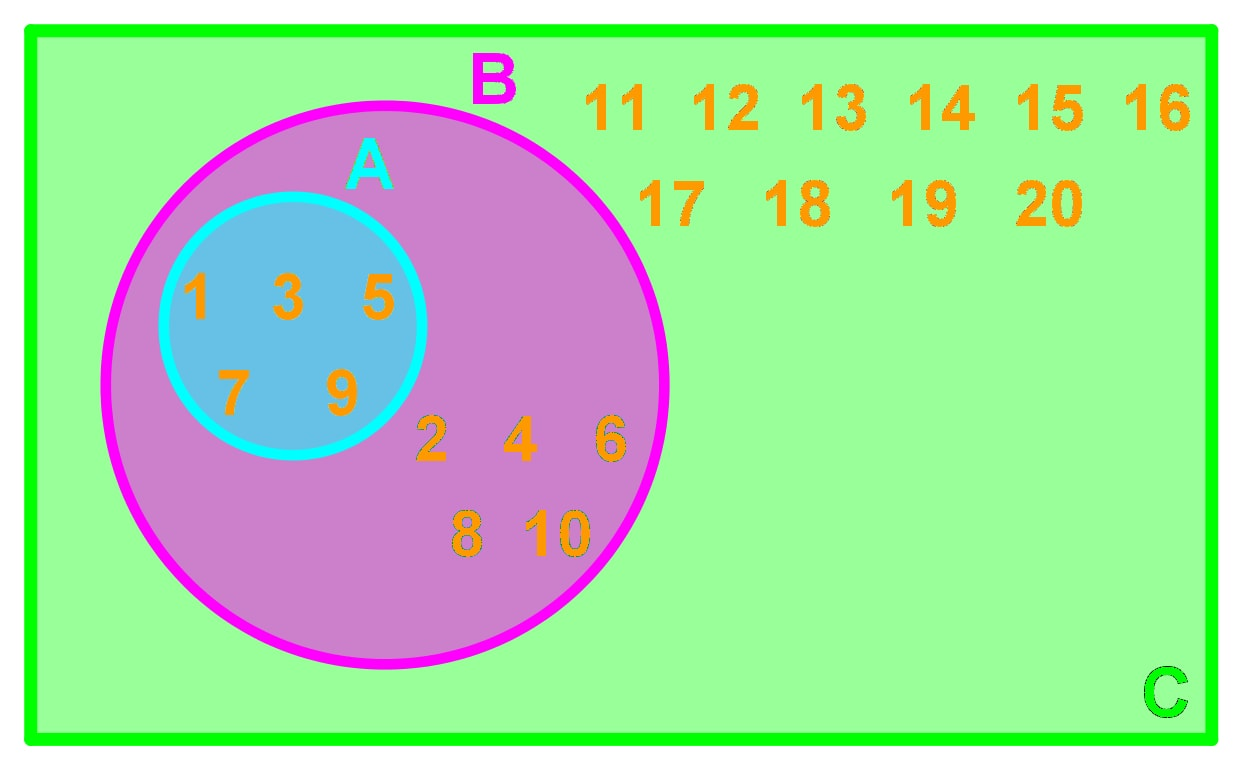# Set notation#### Everything You Need in One Place

Homework problems? Exam preparation? Trying to grasp a concept or just brushing up the basics? Our extensive help & practice library have got you covered.#### Learn and Practice With Ease

Our proven video lessons ease you through problems quickly, and you get tonnes of friendly practice on questions that trip students up on tests and finals.#### Instant and Unlimited Help

Our personalized learning platform enables you to instantly find the exact walkthrough to your specific type of question. Activate unlimited help now!##### Intros
###### Lessons
1. Set Notations Overview: Definitions and Terms
##### Examples
###### Lessons
1. Drawing Venn Diagrams With Sets

Consider the following information:

• $A$ = {1, 2, 3}
• $B$ = {3, 4, 5}
• Universal Set $U$ = {1, 2, 3, 4, 5, 6, 7}

Draw a Venn Diagram describing the 3 sets.

1. Consider the following information:

• $A$ = {1, 2, 3}
• $B$ = {4, 5, 6}
• Universal Set $U$ = {1, 2, 3, 4, 5, 6, 7}

Draw a Venn Diagram describing the 3 sets.

1. Understanding How to Use Set Notation
Consider the following information:
• Universal set $U$ = {0, 1, 2, 3, 4, 5,...}
• Set $N$ = {all natural numbers}
• Set $A$ = {0}
• Set $B$ = { }
1. Is set $N$ a finite set or an infinite set? What about set $B$ ?
2. List all disjoint sets, if any.
3. Determine $n(N)$ , $n(A)$ if possible.
4. Patsy made a statement saying that $n(A)=n(B)$ . Is this true?
5. Is the statement $N \subset U$ true?
2. Consider the following Venn Diagram:
• Universal set $U = \{\mathrm{archery, eating, chess, darts,soccer, basketball, football, volleyball, tennis, badminton}\}$
• Set $A = \{\mathrm{archery, eating, chess, darts}\}$
• Set $B = \{\mathrm{soccer, basketball, football, volleyball}\}$
1. Explain what the sets $A,B$ and $U$ represent.
2. List all disjoint sets, if any.
3. List all the elements of $B'$ .
4. Show that $n(A)+n(A')=n(U)$ .
3. Consider the following Venn Diagram:1. What is the universal set?
2. List all the elements in set $A$ and $B$.
3. Find a subset for set $B$.
4. List all disjoint sets, if any.
5. Find $n(A)$, $n(B)$, and $n(C)$.
6. Is set $C$ a finite set?
4. Drawing and Interpreting Venn Diagrams

Consider the following information:

• Universal Set $U =$ $\mathrm\{-10, -9, -8, -7, -6, -5, -4, -3, -2, -1, 0, 1, 2, 3, 4, 5, 6, 7, 8, 9, 10\}$
• Set $A$ = {positive odd number up to 10}
• Set $B$ = {positive even number up to 10}
• Set $C$ = {0}
1. Draw a Venn diagram
2. List all disjoint sets, if any.
3. Find $n(A)$, $n(B)$, and $n(C)$.
###### Topic Notes

In this lesson, we will learn:

• Drawing Venn Diagrams With Sets
• Understanding How to Use Set Notation
• Drawing and Interpreting Venn Diagrams

Notes:

Here are some terms that we need to know for set notations:

Set: A list of objects or numbers.

Element: An object or a number in a set.

n($A$): The number of elements in set $A$.

Subset: A set where all its elements belong to another set.

Universal Set: A set of all elements in a particular context.

Empty Set: A set with no elements.

Disjoint: Two or more sets that do not have any elements in common.

Mutually Exclusive: Two or more events that cannot happen simultaneously.

Finite Set: A set with a finite number of elements.

Infinite Set: A set with an infinite number of elements.

Complement: The list of remaining elements in the universal set that is not in the mentioned set. If $B$ is a set. Then we defined the complement to be $B'$ or $\overline{B}$.